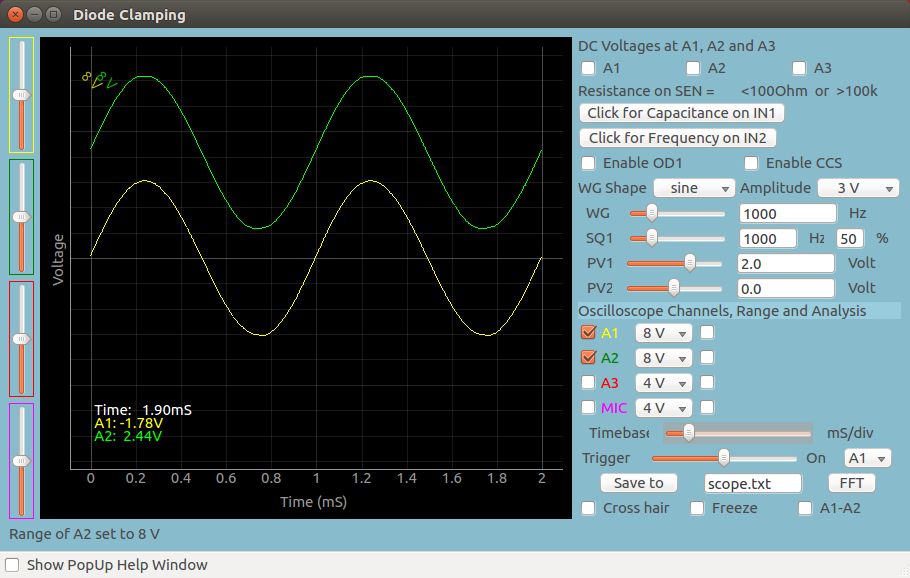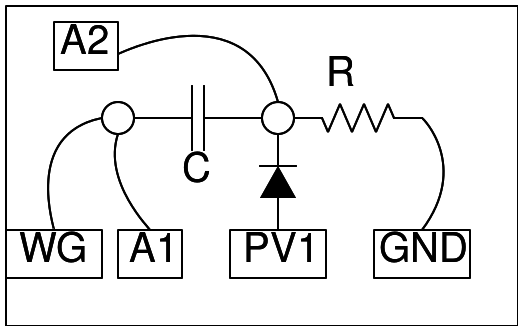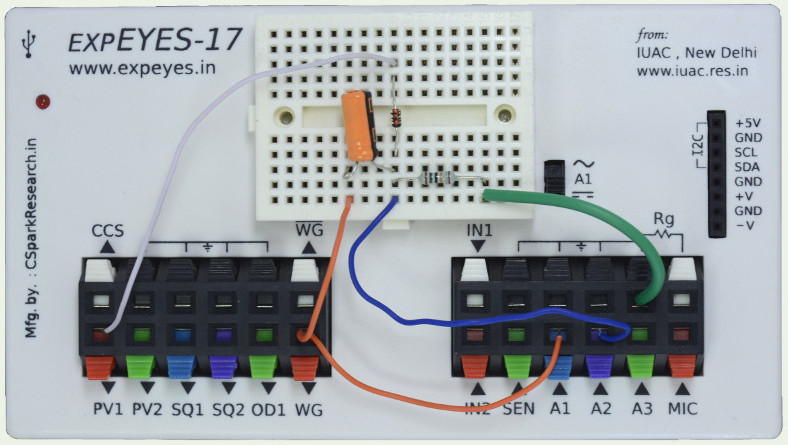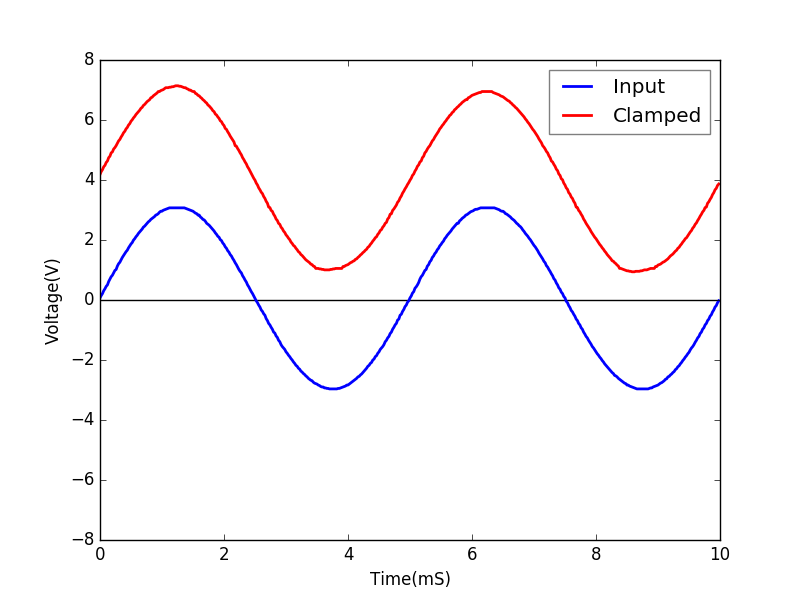# Diode Clamping

## Clamp AC waveforms using a 4148 diodeBrief Summary
Diodes can be used to clamp AC waveforms... a technique which essentially shifts the entire waveform up or down. You will need the waveform generators and oscilloscope of ExpEYES, as well as a voltage output(PV1). and a diode.

## Quick Start

The waveform generator WG is set to give a sine wave of 1kHz. It is monitored by oscilloscope channel A1. The signal after the 1uF capacitor is monitored by A2. The DC voltage to clamp the sine wave is given from PV1, via a diode.

Set the voltage range of A2 to 16 volts, because the voltage after clamping at 5 volts DC could be more that 8 volts.## Screenshot of the UI

Screen shot of the oscilloscope program showing inputs and output of Positive Diode Clamping. 1N4148 diode at 1000Hz. Load is the 1MOhm input impedance of channel A2.## Exercises

• Play with PV1 values, and note down the shifting level and PV1 values. What is the gap between these two. Is it consistent for a particular type of diode?
• Try different diodes.
• Reverse the polarity of the diode. What effect does it have?

## Write Python Code

This experiment can also be done by running this Python Code.

``````import eyes17.eyes
p = eyes17.eyes.open()

from pylab import *

p.set_sine(200)
p.set_pv1(1.35)
t,v, tt,vv = p.capture2(500, 20) # captures A1 and A2

xlabel('Time(mS)') ylabel('Voltage(V)')
plot([0,10], [0,0], 'black')
ylim([-4,4])

plot(t,v,linewidth = 2, color = 'blue')
plot(tt, vv, linewidth = 2, color = 'red')
show()
``````